swarmchart3

3-D swarm scatter chart

Description

Vector Data

example

swarmchart3(x,y,z) displays a 3-D swarm chart, which is a scatter plot with the points offset (jittered) in the x- and y-dimensions. The points form distinct shapes, and the outline of each shape is similar to a violin plot. 3-D swarm charts help you to visualize discrete (x,y) data with the distribution of the z data. At each (x,y) location, the points are jittered based on the kernel density estimate of z.

example

swarmchart3(x,y,z,sz) specifies the marker sizes. To plot all the markers with the same size, specify sz as a scalar. To plot the markers with different sizes, specify sz as a vector that is the same size as x, y, and z.

example

swarmchart3(x,y,z,sz,c) specifies the marker colors. To plot all the markers with the same color, specify c as a color name or an RGB triplet. To assign a different color to each marker, specify a vector the same size as x, y, and z. Alternatively, you can specify a three-column matrix of RGB triplets. The number of rows in the matrix must match the length of x, y, and z.

example

swarmchart3(___,mkr) specifies a different marker than the default marker, which is a circle. Specify mkr after all the arguments in any of the previous syntaxes.

example

swarmchart3(___,'filled') fills in the markers. Specify the 'filled' option after all the arguments in any of the previous syntaxes.

Table Data

example

swarmchart3(tbl,xvar,yvar,zvar) plots the variables xvar, yvar, and zvar from the table tbl. To plot one data set, specify one variable each for xvar, yvar, and zvar. To plot multiple data sets, specify multiple variables for at least one of those arguments. The arguments that specify multiple variables must specify the same number of variables.

example

swarmchart3(tbl,xvar,yvar,zvar,'filled') plots the specified variables from the table with filled circles.

example

swarmchart3(ax,___) displays the swarm chart in the target axes. Specify the axes before all the arguments in any of the previous syntaxes.

swarmchart3(___,Name,Value) specifies additional properties for the swarm chart using one or more Name,Value arguments. For example:

• swarmchart3(x,y,z,'LineWidth',2) creates a swarm chart with 2-point marker outlines.

• swarmchart3(tbl,'MyX','MyY','MyZ','ColorVariable','MyColors') creates a swarm chart from data in a table, and customizes the marker colors using data from the table.

For a list of properties, see Scatter Properties.

example

s = swarmchart3(___) returns the Scatter object. Use s to modify properties of the chart after creating it. For a list of properties, see Scatter Properties.

Examples

collapse all

Read the BicycleCounts.csv data set into a timetable called tbl. This data set contains bicycle traffic data over a period of time. Display the first five rows of tbl.

tbl(1:5,:)
ans=5×5 table
Timestamp              Day         Total    Westbound    Eastbound
___________________    _____________    _____    _________    _________

2015-06-24 00:00:00    {'Wednesday'}     13          9            4
2015-06-24 01:00:00    {'Wednesday'}      3          3            0
2015-06-24 02:00:00    {'Wednesday'}      1          1            0
2015-06-24 03:00:00    {'Wednesday'}      1          1            0
2015-06-24 04:00:00    {'Wednesday'}      1          1            0

Create a vector x with the day name from each observation.

daynames = ["Sunday" "Monday" "Tuesday" "Wednesday" "Thursday" "Friday" "Saturday"];
x = categorical(tbl.Day,daynames);

Create a categorical vector y containing the values "pm" or "am" according to the time for each observation in the table. Create vector z of eastbound traffic data. Then create a swarm chart of x, y, and z. The chart shows the data distributions for each morning and evening of the week.

ispm = tbl.Timestamp.Hour < 12;
y = categorical;
y(ispm) = "pm";
y(~ispm) = "am";
z= tbl.Eastbound;
swarmchart3(x,y,z);Create vector x as a combination of zeros and ones, and create y as a vector containing all ones. Create z as a vector of squared random numbers. Then create a swarm chart of x, y, and z, and specify the size marker size as 5.

x = [zeros(1,500) ones(1,500)];
y = ones(1,1000);
z = randn(1,1000).^2;
swarmchart3(x,y,z,5);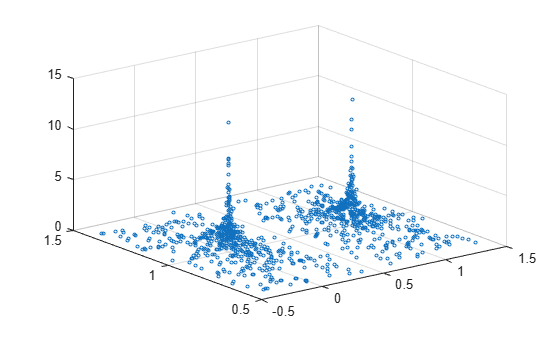Create vector x as a combination of zeros and ones, and create y as a vector containing all ones. Create z as a vector of squared random numbers. Then create a swarm chart of x, y, and z, and specify the point ('.') marker symbol.

x = [zeros(1,500) ones(1,500)];
y = ones(1,1000);
z = randn(1,1000).^2;
swarmchart3(x,y,z,'.');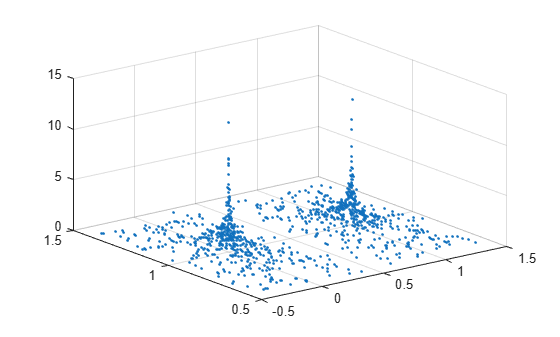Create vector x containing a combination of zeros and ones, and create y containing a random combination of ones and twos. Create z as a vector of squared random numbers. Specify the colors for the markers by creating vector c as the square root of z. Then create a swarm chart of x, y, and z. Set the marker size to 50 and specify the colors as c. The values in c index into the figure's colormap. Use the 'filled' option to fill the markers with color instead of displaying them as hollow circles.

x = [zeros(1,500) ones(1,500)];
y = randi(2,1,1000);
z = randn(1,1000).^2;
c = sqrt(z);
swarmchart3(x,y,z,50,c,'filled');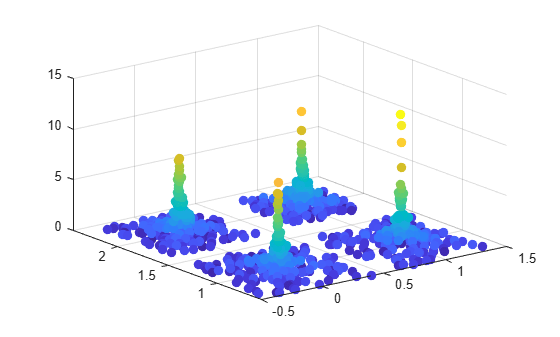Create vector x containing a combination of zeros and ones, and create y containing a random combination of the numbers one through four. Create z as a vector of squared random numbers. Then create a swarm chart of x, y, and z by calling the swarmchart function with a return argument that stores the Scatter object. Add x- and y-axis labels so you can see the effect of changing the jitter properties in each dimension.

x = [zeros(1,500) ones(1,500)];
y = randi(4,1,1000);
z = randn(1,1000).^2;
s = swarmchart3(x,y,z);
xlabel('X')
ylabel('Y')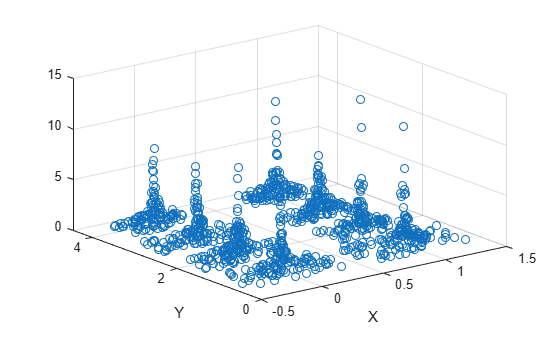Change the shapes of the clusters of points by setting the jitter properties on the Scatter object. In the x dimension, specify uniform random jitter, and change the jitter width to 0.5 data units. In the y dimension, specify normal random jitter, and change the jitter width to 0.1 data units. The spacing between points does not exceed the jitter width you specify.

s.XJitter = 'rand';
s.XJitterWidth = 0.5;
s.YJitter = 'randn';
s.YJitterWidth = 0.1;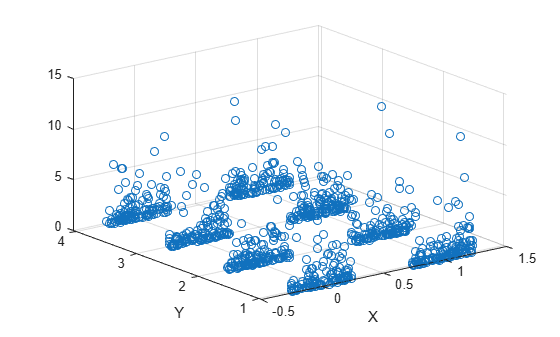A convenient way to plot data from a table is to pass the table to the swarm3 function and specify the variables you want to plot. For example, create a table with four variables of random numbers, and plot the X, Y1, and Z variables. By default, the axis labels match the variable names.

tbl = table(randi(2,100,1),randi(2,100,1),randi([10 11],100,1), ...
randn(100,1),'VariableNames',{'X','Y1','Y2','Z'});

swarmchart3(tbl,'X','Y1','Z')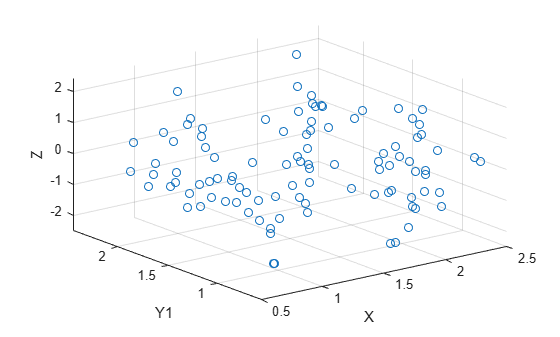You can also plot multiple variables at the same time. For example, plot Y1 and Y2 on the y-axis by specifying the yvar argument as the cell array {'Y1','Y2'}. Then add a legend. The legend labels match the variable names.

swarmchart3(tbl,'X',{'Y1','Y2'},'Z')
legendOne way to plot data from a table and customize the colors and marker sizes is to set the ColorVariable and SizeData properties. You can set these properties as name-value arguments when you call the swarmchart3 function, or you can set them on the Scatter object later.

For example, create a table with four variables of random numbers, and plot the X, Y, and Z variables with filled markers. Vary the marker colors by specifying the ColorVariable name-value argument. Return the Scatter object as s, so you can set other properties later.

tbl = table(randi(2,100,1),randn(100,1),randn(100,1),randn(100,1), ...
'VariableNames',{'X','Y','Z','Colors'});

s = swarmchart3(tbl,'X','Y','Z','filled','ColorVariable','Colors');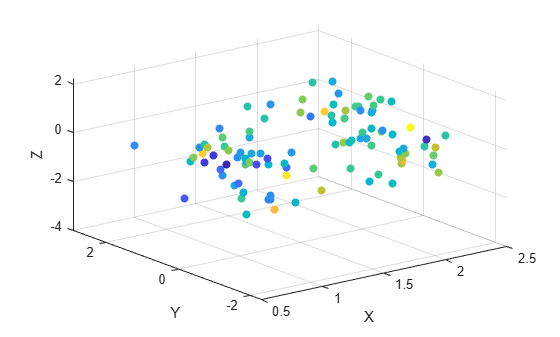Change the marker sizes to 100 points by setting the SizeData property.

s.SizeData = 100;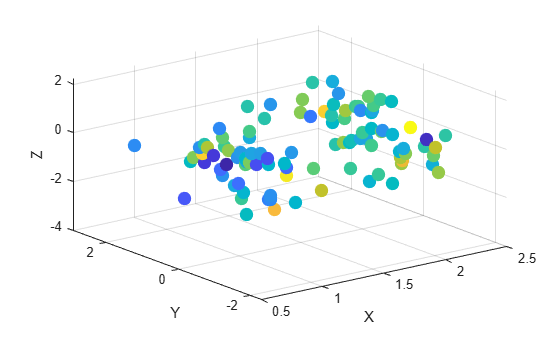Read the BicycleCounts.csv data set into a timetable called tbl. This data set contains bicycle traffic data over a period of time. Display the first five rows of tbl.

tbl(1:5,:)
ans=5×5 table
Timestamp              Day         Total    Westbound    Eastbound
___________________    _____________    _____    _________    _________

2015-06-24 00:00:00    {'Wednesday'}     13          9            4
2015-06-24 01:00:00    {'Wednesday'}      3          3            0
2015-06-24 02:00:00    {'Wednesday'}      1          1            0
2015-06-24 03:00:00    {'Wednesday'}      1          1            0
2015-06-24 04:00:00    {'Wednesday'}      1          1            0

Create vector x with the days names for each observation. Create a categorical vector y containing the values "pm" or "am" according to the time for each observation in the table. Define ze as a vector of eastbound traffic data, and define zw as a vector of westbound traffic data.

daynames = ["Sunday" "Monday" "Tuesday" "Wednesday" "Thursday" "Friday" "Saturday"];
x = categorical(tbl.Day,daynames);
ispm = tbl.Timestamp.Hour<12;
y = categorical;
y(ispm) = 'pm';
y(~ispm) = 'am';
ze = tbl.Eastbound;
zw = tbl.Westbound;

Create a tiled chart layout in the 'flow' tile arrangement, so that the axes fill the available space in the layout. Call the nexttile function to create an axes object and return it as ax1. Then create a swarm chart of the eastbound data by passing ax1 to the swarmchart function.

tiledlayout('flow')
ax1=nexttile;
swarmchart3(ax1,x,y,ze,'.');Repeat the process to create a second axes object and a swarm chart for the westbound traffic.

ax2 = nexttile;
z = tbl.Westbound;
swarmchart3(ax2,x,y,zw,'.');Input Arguments

collapse all

x-coordinates, specified as a numeric scalar or a vector the same size as y and z.

Data Types: single | double | int8 | int16 | int32 | int64 | uint8 | uint16 | uint32 | uint64 | categorical

y-coordinates, specified as a numeric scalar or a vector the same size as x and z.

Data Types: single | double | int8 | int16 | int32 | int64 | uint8 | uint16 | uint32 | uint64 | categorical

z-coordinates, specified as a numeric scalar or a vector the same size as x and y.

Data Types: single | double | int8 | int16 | int32 | int64 | uint8 | uint16 | uint32 | uint64 | datetime | duration

Marker size in points, specified in one of these forms:

• Numeric scalar — Plot all markers with equal size.

• Row or column vector — Use different sizes for each marker. The length of sz must equal the length of x, y, and z.

• [] — Use the default size of 36 points.

Marker color, specified in one of these forms:

• RGB triplet or color name — Plot all the markers with the same color. An RGB triplet is a three-element row vector whose elements specify the intensities of the red, green, and blue components of the color. The intensities must be in the range [0,1]. Alternatively, you can specify a color name from the table below.

• Three column matrix of RGB triplets — Use different colors for each marker. Each row of the matrix specifies an RGB triplet color for the corresponding marker. The number of rows must equal the length of x, y, and z.

• Vector — Use different colors for each marker. The values in c index into the current colormap, and they cover the full range of the colormap. The length of c must equal the length of x, y, and z. To change the colormap, use the colormap function.

Color NameDescriptionEquivalent RGB Triplet
'red' or 'r'Red[1 0 0]
'green' or 'g'Green[0 1 0]
'blue' or 'b'Blue[0 0 1]
'yellow' or 'y'Yellow[1 1 0]
'magenta' or 'm'Magenta[1 0 1]
'cyan' or 'c'Cyan[0 1 1]
'white' or 'w'White[1 1 1]
'black' or 'k'Black[0 0 0]

Marker type, specified as one of the values listed in this table.

MarkerDescriptionResulting Marker
'o'Circle'+'Plus sign'*'Asterisk'.'Point'x'Cross'_'Horizontal line'|'Vertical line's'Square'd'Diamond'^'Upward-pointing triangle'v'Downward-pointing triangle'>'Right-pointing triangle'<'Left-pointing triangle'p'Pentagram'h'HexagramOption to fill the interior of the markers, specified as 'filled'. Use this option with markers that have a face, for example, 'o' or 'square'. Markers that do not have a face and contain only edges do not render at all ('+', '*', '.', and 'x').

The 'filled' option sets the MarkerFaceColor property of the Scatter object to 'flat' and the MarkerEdgeColor property to 'none'. In this case, MATLAB® draws the marker faces, but not the edges.

Source table containing the data to plot. Specify this argument as a table or a timetable.

Table variables containing the x-coordinates, specified as one or more table variable indices.

Specifying Table Indices

Use any of the following indexing schemes to specify the desired variable or variables.

Indexing SchemeExamples

Variable names:

• A character vector or a string scalar.

• A cell array or string vector.

• 'A' or "A" — A variable called A

• {'A','B'} or ["A","B"] — Two variables called A and B

Variable numbers:

• An index number that refers to the location of a variable in the table.

• A vector of numbers.

• 3 — The third variable from the table

• [2 3] — The second and third variables from the table

Logical vector:

• An n-element vector logical vector, where logical 1 (true) values indicate the desired variables.

• Optionally, omit the trailing false values after the last true value.

• [false false true false] — The third variable from an m-by-4 table

• [false false true] — The third variable from any size table

• [false true true] — The second and third variables from any size table

Variable type:

• A vartype command that selects table variables of a specified type.

• vartype('categorical') — All the variables containing categorical values

The table variables you specify can contain numeric, categorical, datetime, or duration values.

To plot one data set, specify one variable for xvar, one variable for yvar, and one variable for zvar. For example, create a table with four variables of normally distributed random values. Plot the X, Y1, and Z variables.

tbl = table(randn(100,1),randn(100,1),randn(100,1)+5,randn(100,1), ...
'VariableNames',{'X','Y1','Y2','Z'});

swarmchart3(tbl,'X','Y1','Z')

To plot multiple data sets together, specify multiple variables for at least one of xvar, yvar, or zvar. If you specify multiple variables for more than one argument, the number of variables must be the same for each of those arguments.

For example, plot the X variable on the x-axis, the Y1 and Y2 variables on the y-axis, and the Z variable on the z-axis.

swarmchart3(tbl,'X',{'Y1','Y2'},'Z')

You can also use different indexing schemes for xvar, yvar, and zvar. For example, specify xvar as a variable name, yvar as an index number, and zvar as a logical vector.

swarmchart3(tbl,'X',2,[false false true])

Table variables containing the y-coordinates, specified as one or more table variable indices.

Specifying Table Indices

Use any of the following indexing schemes to specify the desired variable or variables.

Indexing SchemeExamples

Variable names:

• A character vector or a string scalar.

• A cell array or string vector.

• 'A' or "A" — A variable called A

• {'A','B'} or ["A","B"] — Two variables called A and B

Variable numbers:

• An index number that refers to the location of a variable in the table.

• A vector of numbers.

• 3 — The third variable from the table

• [2 3] — The second and third variables from the table

Logical vector:

• An n-element vector logical vector, where logical 1 (true) values indicate the desired variables.

• Optionally, omit the trailing false values after the last true value.

• [false false true false] — The third variable from an m-by-4 table

• [false false true] — The third variable from any size table

• [false true true] — The second and third variables from any size table

Variable type:

• A vartype command that selects table variables of a specified type.

• vartype('categorical') — All the variables containing categorical values

The table variables you specify can contain numeric, categorical, datetime, or duration values.

To plot one data set, specify one variable for xvar, one variable for yvar, and one variable for zvar. For example, create a table with four variables of normally distributed random values. Plot the X, Y1, and Z variables.

tbl = table(randn(100,1),randn(100,1),randn(100,1)+5,randn(100,1), ...
'VariableNames',{'X','Y1','Y2','Z'});

swarmchart3(tbl,'X','Y1','Z')

To plot multiple data sets together, specify multiple variables for at least one of xvar, yvar, or zvar. If you specify multiple variables for more than one argument, the number of variables must be the same for each of those arguments.

For example, plot the X variable on the x-axis, the Y1 and Y2 variables on the y-axis, and the Z variable on the z-axis.

swarmchart3(tbl,'X',{'Y1','Y2'},'Z')

You can also use different indexing schemes for xvar, yvar, and zvar. For example, specify xvar as a variable name, yvar as an index number, and zvar as a logical vector.

swarmchart3(tbl,'X',2,[false false true])

Table variables containing the z-coordinates, specified as one or more table variable indices.

Specifying Table Indices

Use any of the following indexing schemes to specify the desired variable or variables.

Indexing SchemeExamples

Variable names:

• A character vector or a string scalar.

• A cell array or string vector.

• 'A' or "A" — A variable called A

• {'A','B'} or ["A","B"] — Two variables called A and B

Variable numbers:

• An index number that refers to the location of a variable in the table.

• A vector of numbers.

• 3 — The third variable from the table

• [2 3] — The second and third variables from the table

Logical vector:

• An n-element vector logical vector, where logical 1 (true) values indicate the desired variables.

• Optionally, omit the trailing false values after the last true value.

• [false false true false] — The third variable from an m-by-4 table

• [false false true] — The third variable from any size table

• [false true true] — The second and third variables from any size table

Variable type:

• A vartype command that selects table variables of a specified type.

• vartype('categorical') — All the variables containing categorical values

The table variables you specify can contain numeric, categorical, datetime, or duration values.

To plot one data set, specify one variable for xvar, one variable for yvar, and one variable for zvar. For example, create a table with four variables of normally distributed random values. Plot the X, Y1, and Z variables.

tbl = table(randn(100,1),randn(100,1),randn(100,1)+5,randn(100,1), ...
'VariableNames',{'X','Y1','Y2','Z'});

swarmchart3(tbl,'X','Y1','Z')

To plot multiple data sets together, specify multiple variables for at least one of xvar, yvar, or zvar. If you specify multiple variables for more than one argument, the number of variables must be the same for each of those arguments.

For example, plot the X variable on the x-axis, the Y1 and Y2 variables on the y-axis, and the Z variable on the z-axis.

swarmchart3(tbl,'X',{'Y1','Y2'},'Z')

You can also use different indexing schemes for xvar, yvar, and zvar. For example, specify xvar as a variable name, yvar as an index number, and zvar as a logical vector.

swarmchart3(tbl,'X',2,[false false true])

Target axes, specified as an Axes object. If you do not specify the axes, MATLAB plots into the current axes, or it creates an Axes object if one does not exist.

Name-Value Arguments

Specify optional comma-separated pairs of Name,Value arguments. Name is the argument name and Value is the corresponding value. Name must appear inside quotes. You can specify several name and value pair arguments in any order as Name1,Value1,...,NameN,ValueN.

Example: swarmchart3(randi(2,500,1),randi(2,500,1),randn(500,1),'MarkerFaceColor','red') specifies red filled markers.

Type of jitter (spacing of points) along the x-dimension, specified as one of the following values:

• 'none' — Do not jitter the points.

• 'density' — Jitter the points using the kernel density estimate of y for 2-D charts. If you specify this option in two dimensions for a 3-D chart, the points are jittered based on the kernel density estimate in the third dimension. For example, setting XJitter and YJitter to 'density' uses the kernel density estimate of z.

• 'rand' — Jitter the points randomly with a uniform distribution.

• 'randn' — Jitter points randomly with a normal distribution.

Maximum amount of jitter (offset between points) along the x-dimension, specified as a nonnegative scalar value in data units.

For example, to set the jitter width to 90% of the shortest distance between adjacent points, take the minimum distance between unique values of x and scale by 0.9.

XJitterWidth = 0.9 * min(diff(unique(x)));

Type of jitter (spacing of points) along the y-dimension, specified as one of the following values:

• 'none' — Do not jitter the points.

• 'density' — Jitter the points using the kernel density estimate of x for 2-D charts. If you specify this option in two dimensions for a 3-D chart, the points are jittered based on the kernel density estimate in the third dimension. For example, setting XJitter and YJitter to 'density' uses the kernel density estimate of z.

• 'rand' — Jitter the points randomly with a uniform distribution.

• 'randn' — Jitter points randomly with a normal distribution.

Maximum amount of jitter (offset between points) along the y-dimension, specified as a nonnegative scalar value in data units.

For example, to set the jitter width to 90% of the shortest distance between adjacent points, take the minimum distance between unique values of y and scale by 0.9.

YJitterWidth = 0.9 * min(diff(unique(y)));

Table variable containing the color data, specified as a variable index into the source table.

Specifying the Table Index

Use any of the following indexing schemes to specify the desired variable.

Indexing SchemeExamples

Variable name:

A character vector or a string scalar.

• 'A' or "A" — A variable called A

Variable number:

An index number that refers to the location of a variable in the table.

• 3 — The third variable from the table

Logical vector:

• An n-element vector logical vector, where logical 1 (true) values indicate the desired variable.

• Optionally, omit the trailing false values after the true value.

• [false false true false] — The third variable from an m-by-4 table

• [false false true] — The third variable from any size table

Variable type:

A vartype command that selects table variables of a specified type.

• vartype('double') — The variable containing double values

Specifying Color Data

Specifying the ColorVariable property controls the colors of the markers. The data in the variable controls the marker fill color when the MarkerFaceColor property is set to 'flat'. The data can also control the marker outline color, when the MarkerEdgeColor is set to 'flat'.

The table variable you specify can contain values of any numeric type. The values can be in either of the following forms:

• A column of numbers that linearly map into the current colormap.

• A three-column array of RGB triplets. RGB triplets are three-element vectors whose values specify the intensities of the red, green, and blue components of specific colors. The intensities must be in the range [0,1]. For example, [0.5 0.7 1] specifies a shade of light blue.

When you set the ColorVariable property, MATLAB updates the CData property.

Algorithms

The points in a swarm chart are jittered using uniform random values that are weighted by the Gaussian kernel density estimate of z and the relative number of points at each (x, y) location. This behavior corresponds to the default 'density' setting of the XJitter and YJitter properties on the Scatter object when you call the swarmchart3 function.

The maximum spread of points at each x location is 90% of the smallest distance between adjacent points by default. For example, in the x dimension, the spread is calculated as:

You can control the offset by setting the XJitterWidth and YJitterWidth properties on the Scatter object.